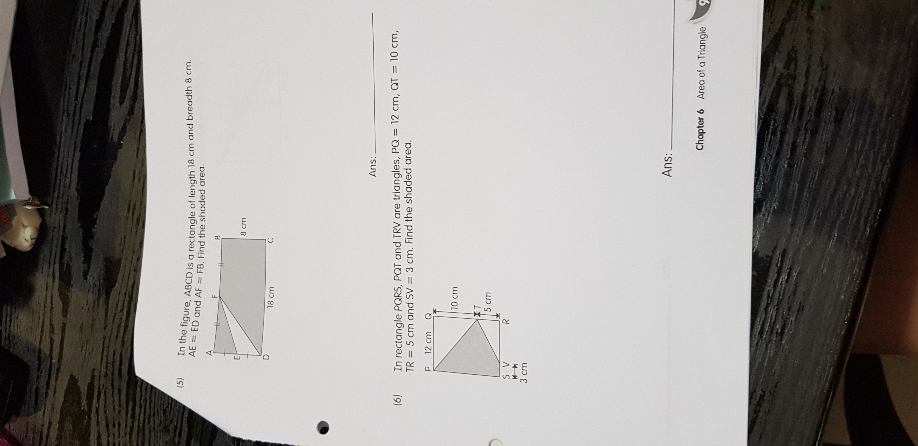# QuestionCan help me solve? Thanks

Source: Geylang Methodist School

1 Answer

# Answer

(5) In the figure, ABCD is a rectangle of length 18 cm and breadth 8 cm. AE = ED and AF = FB. Find the shaded area.

shaded area = 18 x 8 – (1/2) x (8/2) x (18/2) = 126

Ans : 126 sq cm.

n rectangle PQRS, PQT and TRV are triangles, PQ = 12 cm, QT = 10 cm, TR = 5 cm and SV = 3 cm. Find the shaded area.

Drawing a line joining point S and Point T,
the shaded area = (1/2) x (10 + 5) x 12 + (1/2) x 2 x 5 = 97.5
or
shaded area = 12 x (10 + 5) – (1/2) x 12 x 10 – (1/2) x (12 – 3) x 5 = 97.5

Ans : 97.5 sq cm.

0 Replies 0 Likes